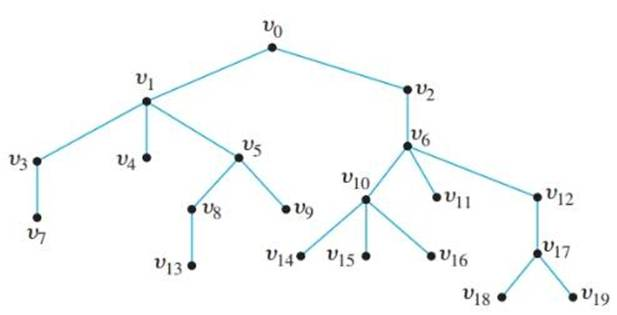Chapter 10.5, Problem 2ES### Discrete Mathematics With Applicat...

5th Edition
EPP + 1 other
ISBN: 9781337694193

#### Solutions

Chapter
Section### Discrete Mathematics With Applicat...

5th Edition
EPP + 1 other
ISBN: 9781337694193
Textbook Problem
28 views

# Consider the tree shown below with root v 0 .a. What is the level of v 8 ? b. What is the level of v 0 ? c. What is the height of this rooted tree?d. What are the children of v 10 ?e. What is the parent of v 5 ? f. What are the siblings of v 1 ?g. What are the descendants of v 12 ? h. How many leaves (terminal vertices) are on the tree?To determine

(a)

What is the level of v8 ?

Explanation

Given info:

Calculation:

The level of a vertex is the length of the path from the root to the vertex.

The root of the tree is v0 (highest vertex in the tree)...

To determine

(b)

What is the level of v0 ?

To determine

(c)

What is the height of this rooted tree?

To determine

(d)

What are the children of v10 ?

To determine

(e)

What is the parent of v5 ?

To determine

(f)

What are the siblings of v1 ?

To determine

(g)

What are the descendants of v12 ?

### Still sussing out bartleby?

Check out a sample textbook solution.

See a sample solution

#### The Solution to Your Study Problems

Bartleby provides explanations to thousands of textbook problems written by our experts, many with advanced degrees!

Get Started